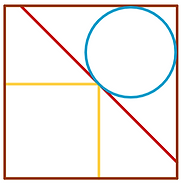Gardner Collection

Sangaku

Sangaku, or “mathematical tablets” in Japanese, are colorful geometric questions or solutions carved on wood.

They were created between the 17th and 19th century by Japanese mathematicians or math enthusiasts who used to hang them in temples or shrines.

Sangaku of

Konnoh Hachimanguo Shrine

(1859)

Source: en.wikipedia.orgSangaku Collection

Each question below is named using the last name of the author combined with the name of the shrine or temple where the sangaku was hung, and sometimes a problem number (if adapted from a sangaku with multiple problems).

Source: Sacred mathematics: Japanese Temple Geometry by Fukagawa Hidetoshi and Tony Rothman (2008).

Shinjun - Katayamahiko #2Kiichi - Abe no Monjuin #10

A circle, a square, and a (blue) equilateral triangle are nestled inside a right triangle. What is the side length of the blue triangle?Atsukuni - Kanzeondo

The two equilateral triangles touch at a midpoint. The radius of the circle is 10. What is the side length of the smaller triangle?Shinjun - Katayamahiko #5

A circle of radius 10 is divided by four segments of length a into five sections of equal area. The middle section is a square of side b. Find a and b.Syouta - Shimizu

A circle of radius 1 and a square of side 2 are placed inside a larger square. Find the side length of the larger square.Nobutomo - Shimizu

A circle of radius 1 and two squares of side 2 are placed next to a larger circle of radius r. What is the length of r